Home > A2C > Chapter 8 > Lesson 8.1.4 > Problem8-64

8-64.
1. Sketch a unit circle. In your circle, sketch in an angle that has: 8-64 HW eTool (Desmos). Homework Help ✎

1. A positive cosine and a negative sine.

2. A sine of −1.

3. A negative cosine and a negative sine.

4. A cosine of about −0.9 and a sine of about 0.4.

5. Could an angle have a sine equal to 0.9 and cosine equal to 0.8? Give an angle or explain why not.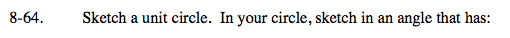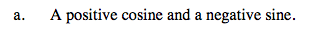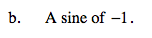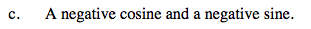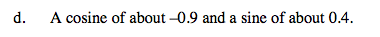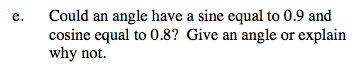Use the eTool below to explore these ideas.
Click the link at right for the full version of the eTool: A2C 8-64 HW eTool